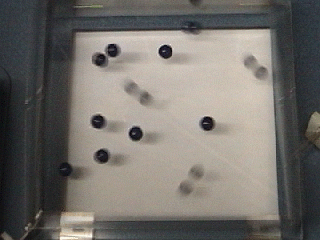### The Molecular Motion Demonstrator (MMD) shows kinetic energy in gaseous atoms.

WATCH VIDEO:

Teachable Topics:

• temperature
• molecular motion
• atomic physics

Theory:

A gas is made up of many molecules, and these molecules are in motion. The MMD is a visual analogy to the motion of gas molecules. The beads in the box represent these molecules.

If the MMD is turned on at low power, the molecules move around at a low speed, on average (as discussed in the "Motion of Molecules in a Gas" page, at a given moment, some molecules are moving quickly while others move slowly). If the power is increased, the molecules move much faster, on average.The group of faster-moving molecules represent a gas at higher temperature. The temperature of a gas depends on the speed of its molecules.

Upon closer examination, the molecules in a gas are all moving at different speeds. Even when the power is low, the speed of the fastest molecule is sometimes greater then that of the slowest molecule when the power is high. This means that the temperature must depend on the average speed of the molecules.

If two different gases are mixed together, they will come into thermal equilibrium. Does this mean that the molecules of the two gases have the same average speed? Putting beads of two different masses in the MMD represents a mix of two different types of gases. Clearly, the larger beads move more slowly. The temperature thus depends not just on the average speed, but on the mass of the gas molecules as wellThe combination of mass and average velocity that determines the temperature of the gas is its average kinetic energy, where the kinetic energy of one particle is T = (1/2) mv2. If a gas has a higher average kinetic energy, it has a higher temperature.

Apparatus:

• Molecular Motion Demonstrator
• 12 medium size blue beads